# R Financial Time Series Plotting

from plot.default to rCharts

# History of R Financial Time Series Plotting

As with all of R, the ability to easily chart financial time series is the result of an iterative progression driven by the collaboration of an extremely dedicated group of open source volunteers. With the release of `rCharts`, I thought it would be interesting to document the timeline of this progression. For each step in the timeline, I will include a link to the source code (svn or github) of the package and a minimal example to demo the "out-of-the-box" capability. In another iteration, I will explore more advanced usage of these functions. Separating the financial time series piece from graphing in general can get murky, and some of the timeline will differ from the timeline of R graphics and the timeline of R time series analysis.

For a much more extensive discussion of time series analysis with R, please see:

Just in case you don't make it to the end,

Thanks to the contributors! I wouldn't be using R if it weren't for you.

First, to build a plot, we need data. Let's see how easy it is to get a time series of financial data in R through `quantmod` `getSymbols()`. The `getSymbols()` function has been a work in progress since December 20, 2006.

``````require(latticeExtra)
require(ggplot2)
require(reshape2)
suppressPackageStartupMessages(
)
require(quantmod)
require(PerformanceAnalytics)
require(xtsExtra)
require(rCharts)

# get S&P 500 data from FRED (St. Louis Fed)
sp500 <- na.omit(
getSymbols(
"SP500",
src = "FRED",
from = "1949-12-31",
auto.assign = FALSE
)
)

# use monthly data
sp500.monthly <- sp500[endpoints(sp500, on ="months")]
``````

## Timeline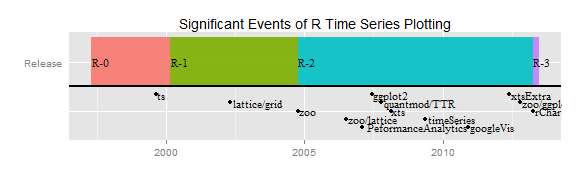### `plot.default` (As Old as Time Itself)

``````# base plot of time series prior to xts
# get the data in data.frame format rather than xts
sp500.df <- data.frame(
index(sp500.monthly),
coredata(sp500.monthly),
stringsAsFactors=FALSE
)
# name columns
colnames( sp500.df ) <- c( "date", "sp500" )
# go back in time to plot.default from the graphics library
graphics::plot.default(
x = sp500.df\$date,
y = sp500.df\$sp500,
type = "l",
xlab = "Date",
ylab = "Closing Value",
main = "S&P 500 (graphics::plot.default)"
)
``````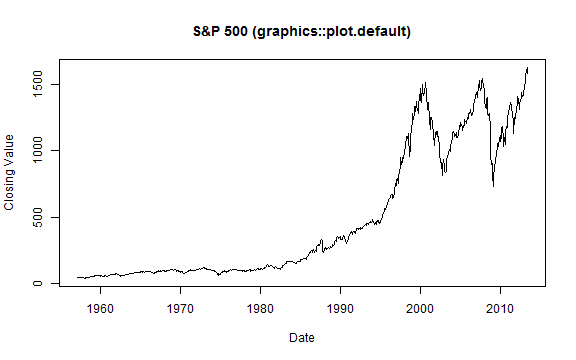### `ts` 1999-08-27

The `ts` package was added in R version 0.65.0 and significantly improved with release 1.5.0 in April 2002. There is a very good discussion of the improvements in Brian Ripley's "Time Series in R 1.5.0" from Volume 2 of R News, June 2002. `plot.ts()` added some nice features, such as the ability to plot multiple/wide time series, specify panels per series, and easily calculate acf, ARIMA,and HoltWinters.

``````stats::plot.ts(
ts(sp500.monthly,
start = c(
as.numeric(format(index(sp500.monthly),"%Y")),
as.numeric(format(index(sp500.monthly),"%m"))
),
frequency = 12
), # some backwards conversion to ts from xts
xlab = "Date",
ylab = "Closing Value",
main = "S&P 500 (stats::plot.ts)"
)
``````### `lattice` and `grid` released with R 1.5.0 2002-04-29

R 1.5.0 was a very important milestone for both graphing and time series analysis with the release of `lattice` (Deepayan Sarkar) and `gri`d (Paul Murrell) and also the improvements in `ts` mentioned above., All of these are covered in Volume 2 of R News, June 2002. `lattice` using `grid` as its platform began an era of aesthetically pleasing and production-quality graphics straight from R.

``````xyplot(
sp500 ~ date,
data = sp500.df,
type = "l",
main = "S&P 500 (lattice::xyplot)"
)
``````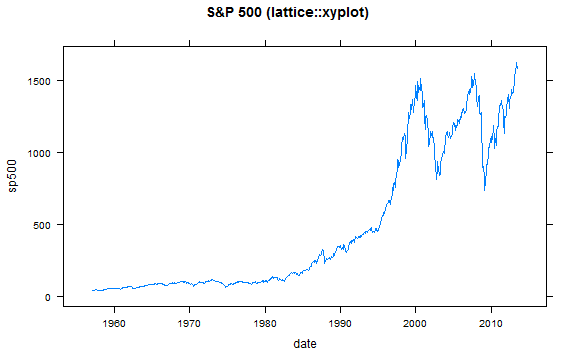### zoo 2004-10-08

`zoo` made it easier to work with irregular time series in R and "bridged the gap." `plot.zoo()` allowed us `plot.ts()` functionality for zoo objects.

``````zoo::plot.zoo(
sp500.monthly,
main = "S&P 500 (zoo::plot.zoo)"
)
``````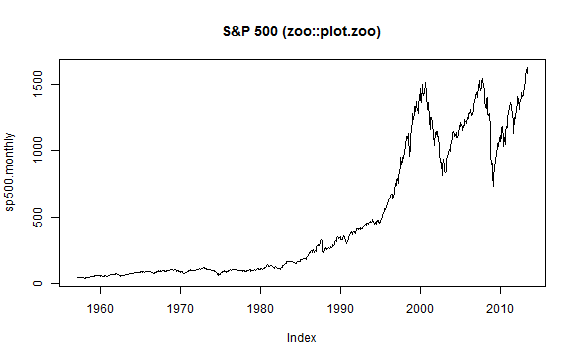### `zoo` Meets `lattice` 2006-07-06

`zoo` adds a very handy `xyplot.zoo()` function so there is no more need to convert `zoo` objects before accessing all the power off `lattice`.

``````# although slightly out of chronology
# I'll also use theEconomist from latticeExtra
asTheEconomist(
xyplot(
sp500.monthly,
scales = list( y = list( rot = 0 ) ),
main = "S&P 500 (lattice::xyplot.xts)"
)
)
``````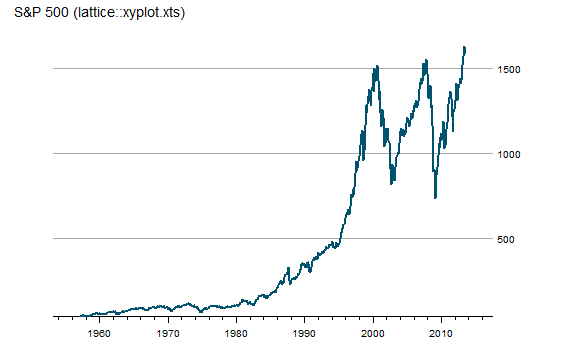### `PerformanceAnalytics` `chart.TimeSerie`s 2007-02-02

`PerformanceAnalytics` addressed many of the graphical patterns necessary for financial performance reporting. `chart.TimeSeries()` and `chart.BarVaR()` serve as the base for functions such as the very useful `charts.PerformanceSummary()` below. In addition to the charts, `PerformanceAnalytics` adds many useful tables and makes both easy and very complicated performance calculations accessible in R. Most of the `PerformanceAnalytics` functions require a `xts` return series rather than price.

``````# 2007-02-02 chart.TimeSeries in PerformanceAnalytics
charts.PerformanceSummary(
ROC(sp500.monthly, n = 1, type = "discrete"),
main = "S&P 500 (PerformanceAnalytice::charts.PerformanceSummary)"
)
``````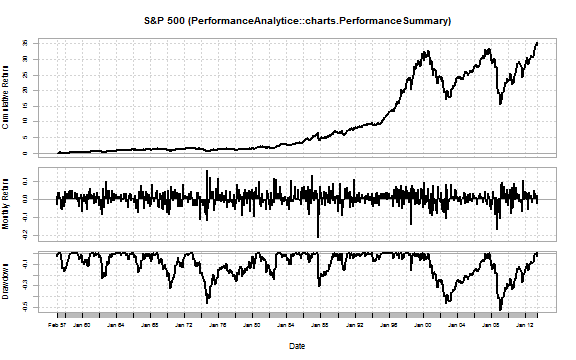### `ggplot2` 2007-06-10

Hadley Wickham's 2005 original `ggplot` was significant, but the 2007 rewrite into `ggplot2` 0.5 completely changed R graphics. Although `ggplot2` is comprehensive and not designed specifically for time series plotting, I include it in the timeline due to both its significant impact on R graphics and its ability to handle dates/times on the x-axis. To use xts with `ggplot2`, a simple conversion to a wide or long format data.frame is necessary.

``````#ggplot2 requires conversion of xts to data.frame
#we will use the data.frame from the plot.default example
ggplot( sp500.df, aes(date) ) +
geom_line( aes( y = sp500 ) ) +
labs( title = "S&P 500 (ggplot2::ggplot)")
``````### `quantmod`/`TTR` `chartSeries` 2007-10-07

`quantmod` and `TTR` were designed to give R technical analysis tools and calculations. The `chartSeries()` function makes OHLC, candlesticks, and bars charts of prices easy. Adding technical analysis, such as Bollinger Bands, RSI, MACD, becomes a couple letter function.

``````chartSeries(
sp500.monthly,
theme = chartTheme("white"),
)
``````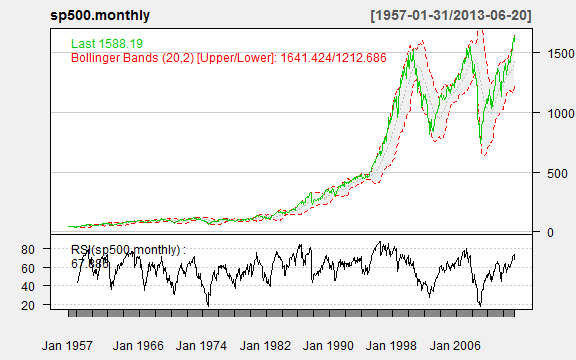Just look how easy it is to zoom.

``````# also easy zooming
zoomChart("1990::")
``````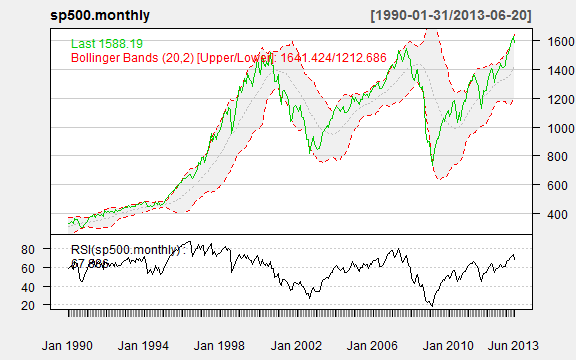### `xts` `plot.xts` 2008-02-17

In 2008, despite the various time series options in R, the world of finance demanded more and Jeff Ryan and Joshua Ulrich responded with `xts`. I strongly recommend reading the `xts` vignette to understand the benefits of `xts`. It is now the standard for financial time series in R. `xts` ported `plot.zoo()` to its own `plot()` method. A `xyplot.xts()` was also provided for use with `lattice`.

``````# 2008-02-17 xts improved zoo and other time series libraries
# http://cran.r-project.org/web/packages/xts/vignettes/xts.pdf
# plot.zoo got ported to plot.xts and little graphing improvement
xts::plot.xts(
sp500.monthly,
ylab = "Closing Value",
main = "S&P 500 (xts::plot.xts)"
)
``````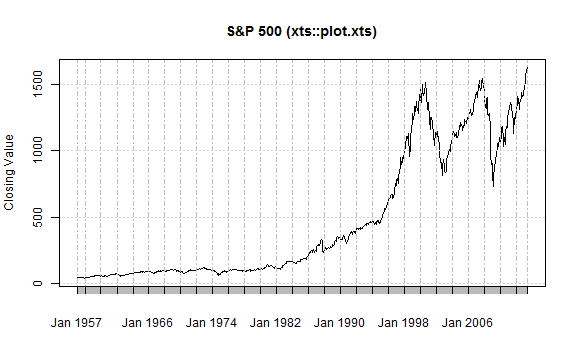### `timeSeries` `plot` 2009-05-17

The `timeSeries` `plot()` method is basically a port of R's `plot.ts()`. It does not significantly add any plotting functionality, but I include it for completeness and since the Rmetrics team offers robust financial analysis through its many R packages that depend on the `timeSeries` object.

``````require(timeSeries)
timeSeries::plot(
timeSeries(sp500.monthly),
main = "S&P 500 (timeseries::plot)"
)
``````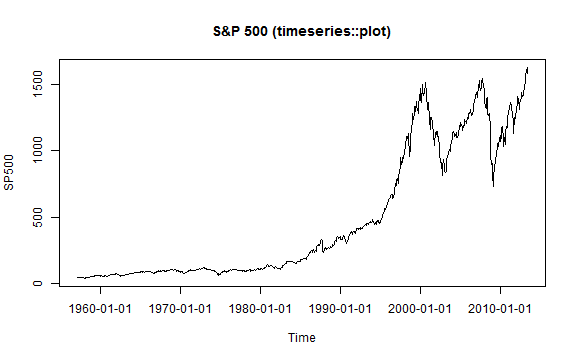### `googleVis` 2010-11-30 and source

`googleVis` adds accessible interactivity to charts using Google Charts. There are a variety of chart types along with very nice animation/storyboard options.

``````g1 <- gvisLineChart(
data = sp500.df,
xvar = "date",
yvar = "sp500",
options = list(
height = 400,
width = 600
)
)
print(g1,"chart")
``````

### xtsExtra plot.xts and barplot.xts 2012-05-30

The Summer 2012 Google Summer of Code project `xtsExtra` by Michael Weylandt sought to improve the `xts` plotting methods as described well in Michael's announcement to R-Sig-Finance.

``````# lots of examples in this post
# http://timelyportfolio.blogspot.com/search/label/plot.xts

#explore barplot.xts to do a chart of annual returns for both indexes
#merge prices
russell2000 <- getSymbols("^RUT", from = "1900-01-01", auto.assign = F)
prices <- merge(sp500,russell2000[,4])
#use endpoints to get annual returns
returns.annual <- as.xts(
apply(
ROC(prices[endpoints(prices,"years")],type="discrete",n=1),
MARGIN = 2,
FUN = na.fill, fill = 0
),
order.by = index(prices[endpoints(prices,"years")])
)
#name columns something a little more clear
colnames(returns.annual) <- c("S&P 500","Russell 2000")

barplot.xts(
returns.annual,
stacked=FALSE,
box="transparent",  #get rid of box surrounding the plot
ylim=c(-0.5,0.5),
ylab=NA,
border=c(brewer.pal(n=11,"BrBG")[c(4,9)]),
col=c(brewer.pal(n=11,"BrBG")[c(4,9)])
)

title(
main="Annual Returns of S&P 500 and Russell 2000 (xtsExtra::barplot.xts)",
outer = TRUE,
font.main = 1,
cex.main = 1.25,
line = -2
)
``````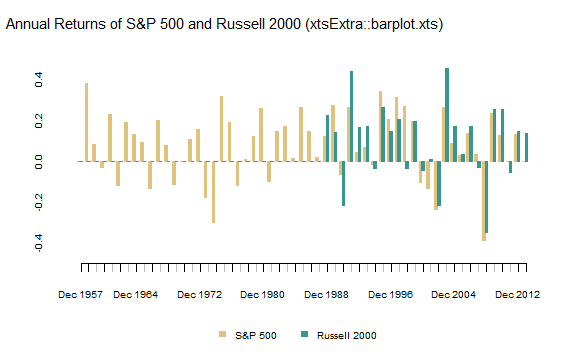### `zoo` meets `ggplot2` with `autoplot.zoo()` 2012-10-14

Similar to `zoo` meets `lattice` above, `autoplot.zoo()` makes plotting `zoo` with `ggplot2` much easier. As the codes shows below, `xts` can also use `autoplot.zoo()` with no explicit transformation.

``````autoplot.zoo(sp500.monthly) +
ggtitle("S&P 500 (zoo::autoplot.zoo)")
``````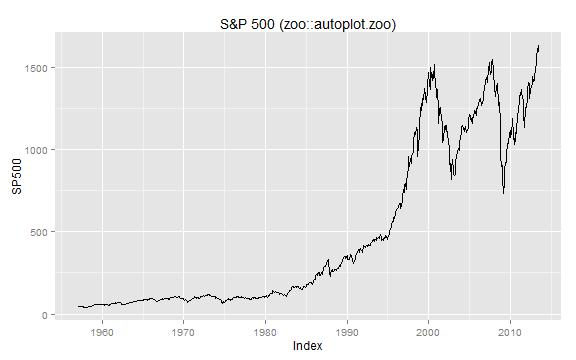### `rCharts` 2013

Although beautiful charts were possible with all the methods above in R, good interactivity was still missing. `rCharts` released in 2013 by Ramnath Vaidyanathan makes interactive charts straight from R with built-in functionality from frameworks built on top of `d3.js`, `raphael`, and other leading javascript libraries. This interactivity offers a whole new level of discovery and exploration previously not available with static graphics. See the examples below. The examples are only minimal examples to demonstrate how much can be done in a few lines of code. For more thorough demos, check out the gallery.

``````# 2013 the world changes with rCharts

# define a function to convert xts wide to long data.frame
xtsMelt <- function(xtsData,metric){
df <- data.frame(index(xtsData),coredata(xtsData),stringsAsFactors=FALSE)
df.melt <- melt(df,id.vars=1)
df.melt <- data.frame(df.melt,rep(metric,NROW(df.melt)))
#little unnecessary housekeeping
df.melt <- df.melt[,c(1,2,4,3)]
colnames(df.melt) <- c("date","indexname","metric","value")
df.melt\$date <- as.Date(df.melt\$date)
#javascript works better when there are no .
#remove troublesome . using modified method from Stack Overflow
i <- sapply(df.melt, is.factor)
df.melt[i] <- lapply(df.melt[i], gsub, pattern="\\.", replacement="")

return(df.melt)
}

sp500.melt <- xtsMelt(
sp500.monthly,
metric = "price"
)

n1 <- nPlot(
value~date,
data = sp500.melt,
group = "indexname",  # even though only one series need to specify group
type = "lineWithFocusChart"
)

n1\$xAxis(
tickFormat=
"#!function(d) {return d3.time.format('%b %Y')(new Date( d ));}!#"
)

n1\$x2Axis(
tickFormat=
"#!function(d) {return d3.time.format('%Y')(new Date( d ));}!#"
)
n1\$print("chart1")
``````

#### morris.js example

``````sp500.df\$date <- format(sp500.df\$date, "%Y-%m-%d")
m1 <- mPlot(
sp500 ~ date,
data = sp500.df,
type = "Line"
)
m1\$set( pointSize = 0 )
m1\$set( hideHover = "auto" )
m1\$print("chart2")
``````

#### rickshaw example

``````#get date in format that rickshaw likes
sp500.df\$date <- as.double(as.POSIXct(as.Date(sp500.df\$date),origin="1970-01-01"))
r1 <- Rickshaw\$new()
r1\$layer(
sp500 ~ date,
data = sp500.df,
type = "line"
)
r1\$set(
slider = TRUE
)
r1\$print("chart3")
``````

#### highcharts example

``````#get in UTC format that works best with Highcharts
sp500.df\$date <- as.numeric(
as.POSIXct(sp500.df\$date, origin="1970-01-01")
) * 1000

h1 <- hPlot(
sp500 ~ date,
data = sp500.df,
type = "line"
)

h1\$xAxis(type = "datetime")
h1\$chart(zoomType = "x")

h1\$plotOptions(
line = list(
marker = list(enabled = F)
)
)

h1\$print("chart5")
``````

## Thanks

Thanks to all the wonderful and diligent contributors who have made R great.